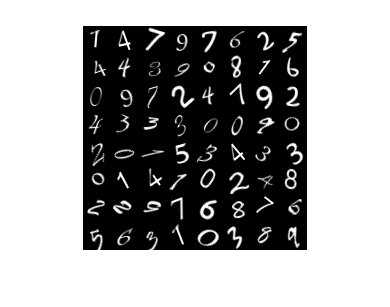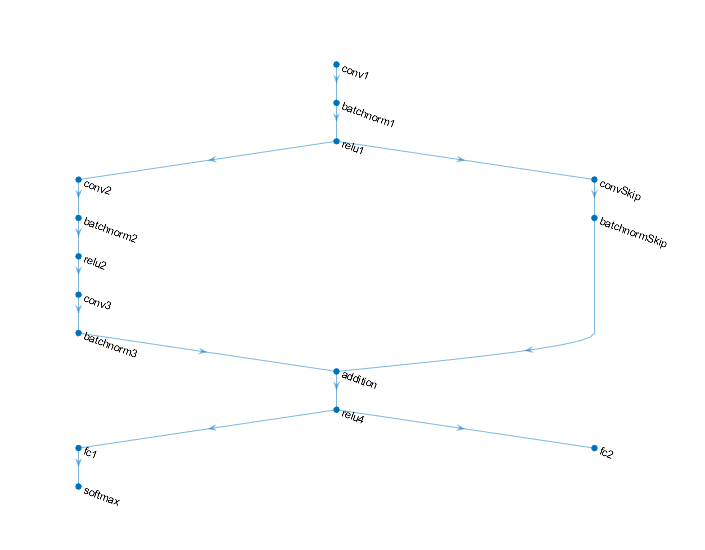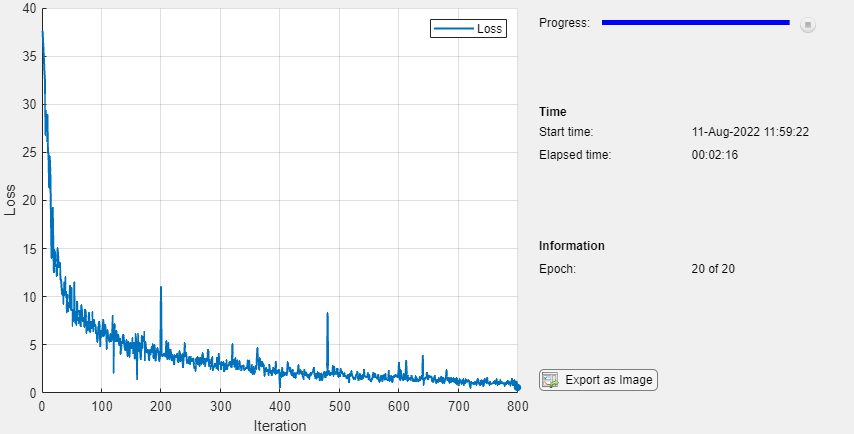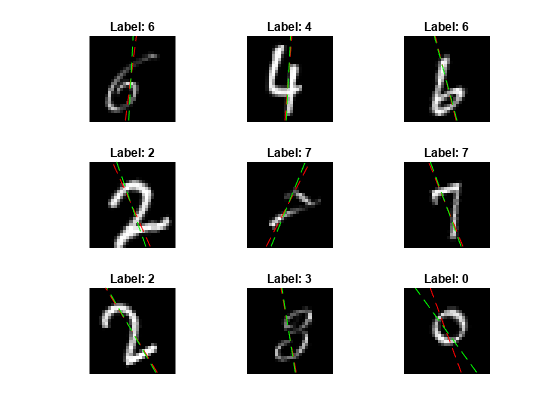Train Network Using Model Function

This example shows how to create and train a deep learning network by using functions rather than a layer graph or a dlnetwork. The advantage of using functions is the flexibility to describe a wide variety of networks. The disadvantage is that you must complete more steps and prepare your data carefully. This example uses images of handwritten digits, with the dual objectives of classifying the digits and determining the angle of each digit from the vertical.

The digitTrain4DArrayData function loads the images, their digit labels, and their angles of rotation from the vertical. Create arrayDatastore objects for the images, labels, and angles, and then use the combine function to make a single datastore that contains all of the training data. Extract the class names and number of nondiscrete responses.

[XTrain,YTrain,anglesTrain] = digitTrain4DArrayData;

dsXTrain = arrayDatastore(XTrain,'IterationDimension',4);
dsYTrain = arrayDatastore(YTrain);
dsAnglesTrain = arrayDatastore(anglesTrain);

dsTrain = combine(dsXTrain,dsYTrain,dsAnglesTrain);

classNames = categories(YTrain);
numClasses = numel(classNames);
numResponses = size(anglesTrain,2);
numObservations = numel(YTrain);

View some images from the training data.

idx = randperm(numObservations,64);
I = imtile(XTrain(:,:,:,idx));
figure
imshow(I)Define Deep Learning Model

Define the following network that predicts both labels and angles of rotation.

• A convolution-batchnorm-ReLU block with 16 5-by-5 filters.

• A branch of two convolution-batchnorm blocks each with 32 3-by-3 filters with a ReLU operation between

• A skip connection with a convolution-batchnorm block with 32 1-by-1 convolutions.

• Combine both branches using addition followed by a ReLU operation

• For the regression output, a branch with a fully connected operation of size 1 (the number of responses).

• For classification output, a branch with a fully connected operation of size 10 (the number of classes) and a softmax operation.Define and Initialize Model Parameters and State

Define the parameters for each of the operations and include them in a struct. Use the format parameters.OperationName.ParameterName where parameters is the struct, OperationName is the name of the operation (for example "conv1") and ParameterName is the name of the parameter (for example, "Weights").

Create a struct parameters containing the model parameters. Initialize the learnable layer weights and biases using the initializeGlorot and initializeZeros example functions, respectively. Initialize the batch normalization offset and scale parameters with the initializeZeros and initializeOnes example functions, respectively.

To perform training and inference using batch normalization layers, you must also manage the network state. Before prediction, you must specify the dataset mean and variance derived from the training data. Create a struct state containing the state parameters. The batch normalization statistics must not be dlarray objects. Initialize the batch normalization trained mean and trained variance states using the zeros and ones functions, respectively.

The initialization example functions are attached to this example as supporting files.

Initialize the parameters for the first convolutional layer.

filterSize = [5 5];
numChannels = 1;
numFilters = 16;

sz = [filterSize numChannels numFilters];
numOut = prod(filterSize) * numFilters;
numIn = prod(filterSize) * numFilters;

parameters.conv1.Weights = initializeGlorot(sz,numOut,numIn);
parameters.conv1.Bias = initializeZeros([numFilters 1]);

Initialize the parameters and state for the first batch normalization layer.

parameters.batchnorm1.Offset = initializeZeros([numFilters 1]);
parameters.batchnorm1.Scale = initializeOnes([numFilters 1]);
state.batchnorm1.TrainedMean = zeros(numFilters,1,'single');
state.batchnorm1.TrainedVariance = ones(numFilters,1,'single');

Initialize the parameters for the second convolutional layer.

filterSize = [3 3];
numChannels = 16;
numFilters = 32;

sz = [filterSize numChannels numFilters];
numOut = prod(filterSize) * numFilters;
numIn = prod(filterSize) * numFilters;

parameters.conv2.Weights = initializeGlorot(sz,numOut,numIn);
parameters.conv2.Bias = initializeZeros([numFilters 1]);

Initialize the parameters and state for the second batch normalization layer.

parameters.batchnorm2.Offset = initializeZeros([numFilters 1]);
parameters.batchnorm2.Scale = initializeOnes([numFilters 1]);
state.batchnorm2.TrainedMean = zeros(numFilters,1,'single');
state.batchnorm2.TrainedVariance = ones(numFilters,1,'single');

Initialize the parameters for the third convolutional layer.

filterSize = [3 3];
numChannels = 32;
numFilters = 32;

sz = [filterSize numChannels numFilters];
numOut = prod(filterSize) * numFilters;
numIn = prod(filterSize) * numFilters;

parameters.conv3.Weights = initializeGlorot(sz,numOut,numIn);
parameters.conv3.Bias = initializeZeros([numFilters 1]);

Initialize the parameters and state for the third batch normalization layer.

parameters.batchnorm3.Offset = initializeZeros([numFilters 1]);
parameters.batchnorm3.Scale = initializeOnes([numFilters 1]);
state.batchnorm3.TrainedMean = zeros(numFilters,1,'single');
state.batchnorm3.TrainedVariance = ones(numFilters,1,'single');

Initialize the parameters for the convolutional layer in the skip connection.

filterSize = [1 1];
numChannels = 16;
numFilters = 32;

sz = [filterSize numChannels numFilters];
numOut = prod(filterSize) * numFilters;
numIn = prod(filterSize) * numFilters;

parameters.convSkip.Weights = initializeGlorot(sz,numOut,numIn);
parameters.convSkip.Bias = initializeZeros([numFilters 1]);

Initialize the parameters and state for the batch normalization layer in the skip connection.

parameters.batchnormSkip.Offset = initializeZeros([numFilters 1]);
parameters.batchnormSkip.Scale = initializeOnes([numFilters 1]);
state.batchnormSkip.TrainedMean = zeros([numFilters 1],'single');
state.batchnormSkip.TrainedVariance = ones([numFilters 1],'single');

Initialize the parameters for the fully connected layer corresponding to the classification output.

sz = [numClasses 6272];
numOut = numClasses;
numIn = 6272;
parameters.fc1.Weights = initializeGlorot(sz,numOut,numIn);
parameters.fc1.Bias = initializeZeros([numClasses 1]);

Initialize the parameters for the fully connected layer corresponding to the regression output.

sz = [numResponses 6272];
numOut = numResponses;
numIn = 6272;
parameters.fc2.Weights = initializeGlorot(sz,numOut,numIn);
parameters.fc2.Bias = initializeZeros([numResponses 1]);

View the struct of the parameters.

parameters
parameters = struct with fields:
conv1: [1×1 struct]
batchnorm1: [1×1 struct]
conv2: [1×1 struct]
batchnorm2: [1×1 struct]
conv3: [1×1 struct]
batchnorm3: [1×1 struct]
convSkip: [1×1 struct]
batchnormSkip: [1×1 struct]
fc1: [1×1 struct]
fc2: [1×1 struct]

View the parameters for the "conv1" operation.

parameters.conv1
ans = struct with fields:
Weights: [5×5×1×16 dlarray]
Bias: [16×1 dlarray]

View the struct of the state.

state
state = struct with fields:
batchnorm1: [1×1 struct]
batchnorm2: [1×1 struct]
batchnorm3: [1×1 struct]
batchnormSkip: [1×1 struct]

View the state parameters for the "batchnorm1" operation.

state.batchnorm1
ans = struct with fields:
TrainedMean: [16×1 single]
TrainedVariance: [16×1 single]

Define Model Function

Create the function model, listed at the end of the example, that computes the outputs of the deep learning model described earlier.

The function model takes the model parameters parameters, the input data dlX, the flag doTraining which specifies whether to model should return outputs for training or prediction, and the network state state. The network outputs the predictions for the labels, the predictions for the angles, and the updated network state.

Create the function modelGradients, listed at the end of the example, that takes the model parameters, a mini-batch of input data dlX with corresponding targets T1 and T2 containing the labels and angles, respectively, and returns the gradients of the loss with respect to the learnable parameters, the updated network state, and the corresponding loss.

Specify Training Options

Specify the training options. Train for 20 epochs with a mini-batch size of 128.

numEpochs = 20;
miniBatchSize = 128;

To monitor the training progress, you can plot the training loss after each iteration. Create the variable plots that contains "training-progress". If you do not want to plot the training progress, then set this value to "none".

plots = "training-progress";

Train Model

Use minibatchqueue to process and manage the mini-batches of images. For each mini-batch:

• Use the custom mini-batch preprocessing function preprocessMiniBatch (defined at the end of this example) to one-hot encode the class labels.

• Format the image data with the dimension labels 'SSCB' (spatial, spatial, channel, batch). By default, the minibatchqueue object converts the data to dlarray objects with underlying type single. Do not add a format to the class labels or angles.

• Train on a GPU if one is available. By default, the minibatchqueue object converts each output to a gpuArray if a GPU is available. Using a GPU requires Parallel Computing Toolbox™ and a supported GPU device. For information on supported devices, see GPU Support by Release (Parallel Computing Toolbox).

mbq = minibatchqueue(dsTrain,...
'MiniBatchSize',miniBatchSize,...
'MiniBatchFcn', @preprocessMiniBatch,...
'MiniBatchFormat',{'SSCB','',''});

For each epoch, shuffle the data and loop over mini-batches of data. At the end of each iteration, display the training progress. For each mini-batch:

• Evaluate the model gradients and loss using dlfeval and the modelGradients function.

• Update the network parameters using the adamupdate function.

trailingAvg = [];
trailingAvgSq = [];

Initialize the training progress plot.

if plots == "training-progress"
figure
lineLossTrain = animatedline('Color',[0.85 0.325 0.098]);
ylim([0 inf])
xlabel("Iteration")
ylabel("Loss")
grid on
end

Train the model.

iteration = 0;
start = tic;

% Loop over epochs.
for epoch = 1:numEpochs

% Shuffle data.
shuffle(mbq)

% Loop over mini-batches
while hasdata(mbq)

iteration = iteration + 1;

[dlX,dlY1,dlY2] = next(mbq);

% Evaluate the model gradients, state, and loss using dlfeval and the

% Update the network parameters using the Adam optimizer.
trailingAvg,trailingAvgSq,iteration);

% Display the training progress.
if plots == "training-progress"
D = duration(0,0,toc(start),'Format','hh:mm:ss');
title("Epoch: " + epoch + ", Elapsed: " + string(D))
drawnow
end
end
endTest Model

Test the classification accuracy of the model by comparing the predictions on a test set with the true labels and angles. Manage the test data set using a minibatchqueue object with the same setting as the training data.

[XTest,YTest,anglesTest] = digitTest4DArrayData;

dsXTest = arrayDatastore(XTest,'IterationDimension',4);
dsYTest = arrayDatastore(YTest);
dsAnglesTest = arrayDatastore(anglesTest);

dsTest = combine(dsXTest,dsYTest,dsAnglesTest);

mbqTest = minibatchqueue(dsTest,...
'MiniBatchSize',miniBatchSize,...
'MiniBatchFcn', @preprocessMiniBatch,...
'MiniBatchFormat',{'SSCB','',''});

To predict the labels and angles of the validation data, loop over the mini-batches and use the model function with the doTraining option set to false. Store the predicted classes and angles. Compare the predicted and true classes and angles and store the results.

doTraining = false;

classesPredictions = [];
anglesPredictions = [];
classCorr = [];
angleDiff = [];

% Loop over mini-batches.
while hasdata(mbqTest)

[dlXTest,dlY1Test,dlY2Test] = next(mbqTest);

% Make predictions using the predict function.
[dlY1Pred,dlY2Pred] = model(parameters,dlXTest,doTraining,state);

% Determine predicted classes.
Y1PredBatch = onehotdecode(dlY1Pred,classNames,1);
classesPredictions = [classesPredictions Y1PredBatch];

% Dermine predicted angles
Y2PredBatch = extractdata(dlY2Pred);
anglesPredictions = [anglesPredictions Y2PredBatch];

% Compare predicted and true classes
Y1Test = onehotdecode(dlY1Test,classNames,1);
classCorr = [classCorr Y1PredBatch == Y1Test];

% Compare predicted and true angles
angleDiffBatch = Y2PredBatch - dlY2Test;
angleDiff = [angleDiff extractdata(gather(angleDiffBatch))];

end

Evaluate the classification accuracy.

accuracy = mean(classCorr)
accuracy = 0.9730

Evaluate the regression accuracy.

angleRMSE = sqrt(mean(angleDiff.^2))
angleRMSE = single
6.6909

View some of the images with their predictions. Display the predicted angles in red and the correct labels in green.

idx = randperm(size(XTest,4),9);
figure
for i = 1:9
subplot(3,3,i)
I = XTest(:,:,:,idx(i));
imshow(I)
hold on

sz = size(I,1);
offset = sz/2;

thetaPred = anglesPredictions(idx(i));
plot(offset*[1-tand(thetaPred) 1+tand(thetaPred)],[sz 0],'r--')

thetaValidation = anglesTest(idx(i));
plot(offset*[1-tand(thetaValidation) 1+tand(thetaValidation)],[sz 0],'g--')

hold off
label = string(classesPredictions(idx(i)));
title("Label: " + label)
endModel Function

The function model takes the model parameters parameters, the input data dlX, the flag doTraining which specifies whether to model should return outputs for training or prediction, and the network state state. The network outputs the predictions for the labels, the predictions for the angles, and the updated network state.

function [dlY1,dlY2,state] = model(parameters,dlX,doTraining,state)

% Convolution
weights = parameters.conv1.Weights;
bias = parameters.conv1.Bias;

% Batch normalization, ReLU
offset = parameters.batchnorm1.Offset;
scale = parameters.batchnorm1.Scale;
trainedMean = state.batchnorm1.TrainedMean;
trainedVariance = state.batchnorm1.TrainedVariance;

if doTraining
[dlY,trainedMean,trainedVariance] = batchnorm(dlY,offset,scale,trainedMean,trainedVariance);

% Update state
state.batchnorm1.TrainedMean = trainedMean;
state.batchnorm1.TrainedVariance = trainedVariance;
else
dlY = batchnorm(dlY,offset,scale,trainedMean,trainedVariance);
end

dlY = relu(dlY);

% Convolution, batch normalization (Skip connection)
weights = parameters.convSkip.Weights;
bias = parameters.convSkip.Bias;
dlYSkip = dlconv(dlY,weights,bias,'Stride',2);

offset = parameters.batchnormSkip.Offset;
scale = parameters.batchnormSkip.Scale;
trainedMean = state.batchnormSkip.TrainedMean;
trainedVariance = state.batchnormSkip.TrainedVariance;

if doTraining
[dlYSkip,trainedMean,trainedVariance] = batchnorm(dlYSkip,offset,scale,trainedMean,trainedVariance);

% Update state
state.batchnormSkip.TrainedMean = trainedMean;
state.batchnormSkip.TrainedVariance = trainedVariance;
else
dlYSkip = batchnorm(dlYSkip,offset,scale,trainedMean,trainedVariance);
end

% Convolution
weights = parameters.conv2.Weights;
bias = parameters.conv2.Bias;

% Batch normalization, ReLU
offset = parameters.batchnorm2.Offset;
scale = parameters.batchnorm2.Scale;
trainedMean = state.batchnorm2.TrainedMean;
trainedVariance = state.batchnorm2.TrainedVariance;

if doTraining
[dlY,trainedMean,trainedVariance] = batchnorm(dlY,offset,scale,trainedMean,trainedVariance);

% Update state
state.batchnorm2.TrainedMean = trainedMean;
state.batchnorm2.TrainedVariance = trainedVariance;
else
dlY = batchnorm(dlY,offset,scale,trainedMean,trainedVariance);
end

dlY = relu(dlY);

% Convolution
weights = parameters.conv3.Weights;
bias = parameters.conv3.Bias;

% Batch normalization
offset = parameters.batchnorm3.Offset;
scale = parameters.batchnorm3.Scale;
trainedMean = state.batchnorm3.TrainedMean;
trainedVariance = state.batchnorm3.TrainedVariance;

if doTraining
[dlY,trainedMean,trainedVariance] = batchnorm(dlY,offset,scale,trainedMean,trainedVariance);

% Update state
state.batchnorm3.TrainedMean = trainedMean;
state.batchnorm3.TrainedVariance = trainedVariance;
else
dlY = batchnorm(dlY,offset,scale,trainedMean,trainedVariance);
end

dlY = dlYSkip + dlY;
dlY = relu(dlY);

% Fully connect, softmax (labels)
weights = parameters.fc1.Weights;
bias = parameters.fc1.Bias;
dlY1 = fullyconnect(dlY,weights,bias);
dlY1 = softmax(dlY1);

% Fully connect (angles)
weights = parameters.fc2.Weights;
bias = parameters.fc2.Bias;
dlY2 = fullyconnect(dlY,weights,bias);

end

The modelGradients function, takes the model parameters, a mini-batch of input data dlX with corresponding targets T1 and T2 containing the labels and angles, respectively, and returns the gradients of the loss with respect to the learnable parameters, the updated network state, and the corresponding loss.

doTraining = true;
[dlY1,dlY2,state] = model(parameters,dlX,doTraining,state);

lossLabels = crossentropy(dlY1,T1);
lossAngles = mse(dlY2,T2);

loss = lossLabels + 0.1*lossAngles;

end

Mini-Batch Preprocessing Function

The preprocessMiniBatch function preprocesses the data using the following steps:

1. Extract the image data from the incoming cell array and concatenate into a numeric array. Concatenating the image data over the fourth dimension adds a third dimension to each image, to be used as a singleton channel dimension.

2. Extract the label and angle data from the incoming cell arrays and concatenate along the second dimension into a categorical array and a numeric array, respectively.

3. One-hot encode the categorical labels into numeric arrays. Encoding into the first dimension produces an encoded array that matches the shape of the network output.

function [X,Y,angle] = preprocessMiniBatch(XCell,YCell,angleCell)

% Extract image data from cell and concatenate
X = cat(4,XCell{:});
% Extract label data from cell and concatenate
Y = cat(2,YCell{:});
% Extract angle data from cell and concatenate
angle = cat(2,angleCell{:});

% One-hot encode labels
Y = onehotencode(Y,1);

end